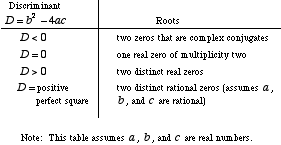index: click on a letter A B C D E F G H I J K L M N O P Q R S T U V W X Y Z A to Z index index: subject areas numbers & symbols sets, logic, proofs geometry algebra trigonometry advanced algebra & pre-calculus calculus advanced topics probability & statistics real world applications multimedia entrieswww.mathwords.com about mathwords website feedback

 Discriminant of a Quadratic The number D = b2 – 4ac determined from the coefficients of the equation ax2 + bx + c = 0. The discriminant reveals what type of roots the equation has. Note: b2 – 4ac comes from the quadratic formula.See also